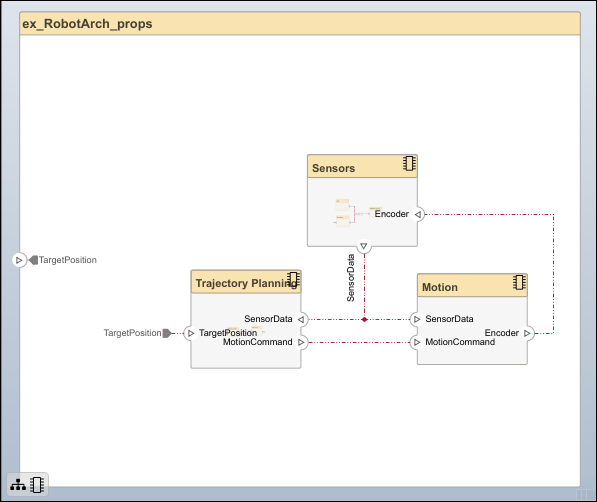## Analyze an Architecture Model with an Analysis Function

With properties specified on model elements, you can use MATLAB® to perform analysis and calculate total cost for all elements within the design. You can then create additional derived requirements for the designers of individual components in the system, such as `Trajectory Planning` or `Sensors`.

Perform static analyses based on element properties to perform data-driven trade studies and verify system requirements. Consider a robot architecture model where total cost is a consideration. For this tutorial, you will use the mobile robot architecture model with properties to perform static analysis.

### Mobile Robot Architecture Model with Properties

This example shows a mobile robot architecture model with stereotypes applied to components and properties defined.### Perform an Analysis

Analyze the total cost for all components in the robot model. This procedure uses the model `ex_RobotArch_props.slx`.

1. Navigate to Modeling > Views > Analysis Model to open the Instantiate Architecture Model dialog.

2. Add an analysis function. In the Analysis function box, enter the function name `ex_RobotArch_analysis` without an extension, and then click thebutton. A MATLAB function file is created and saved with the name `ex_RobotArch_analysis.m`.The analysis function includes constructs that get properties from model elements, given as a template. Modify this template to add the cost of individual elements and obtain total cost for their parent architecture. This function computes the cost for one model element as a total of its own cost and the cost of all of its child components. Copy and paste the function below into your analysis function.

```function ex_RobotArch_analysis(instance,varargin) if instance.isComponent() if instance.hasValue("sysComponent.unitPrice") % Check if price is defined sysComponent_unitPrice = instance.getValue("sysComponent.unitPrice"); for child = instance.Components % Iterate through all components if child.hasValue("sysComponent.unitPrice") comp_price = child.getValue("sysComponent.unitPrice"); sysComponent_unitPrice = sysComponent_unitPrice + comp_price; end end for child = instance.Connectors % Connectors are priced by length if child.hasValue("sysConnector.unitPrice") unitPrice = child.getValue("sysConnector.unitPrice"); length = child.getValue("sysConnector.length"); sysComponent_unitPrice = unitPrice*length + sysComponent_unitPrice; end end instance.setValue("sysComponent.unitPrice",sysComponent_unitPrice) % Set totals end end```
3. Return to the Instantiate Architecture Model screen and click . The Analysis Viewer opens and shows the properties of each model element. The default values for the start of the analysis are taken from the property values you entered when you attached the stereotype to the model and edited their values.

4. In the Analysis section, select `BottomUp` as the iteration method, then click .

The cost of each element is added bottom-up to find the cost of the system. The result is written to the analysis instance and is visible in the Analysis Viewer.The total cost is highlighted in yellow as a computed value on the top row representing the `ex_RobotArch_props` architecture.

## SupportGet trial now